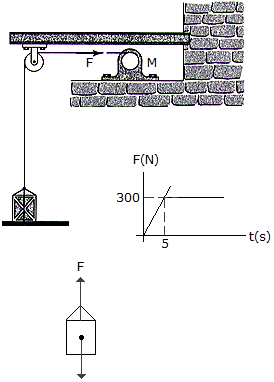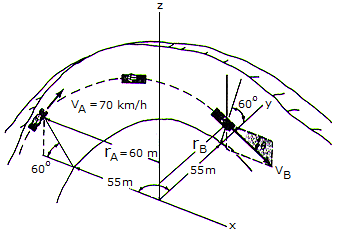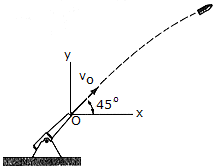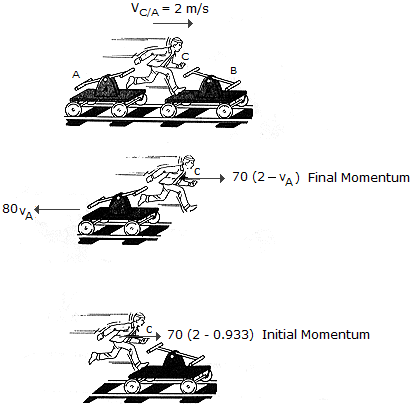# Engineering Mechanics - KOP: Impulse and Momentum

### Exercise :: KOP: Impulse and Momentum - General Questions

• KOP: Impulse and Momentum - General Questions
6.The motor M pulls on the cables with a force F that has a magnitude which varies as shown on the graph. If the 15-kg crate is originally resting on the floor such that the cable tension is zero when the motor is turned on, determine the speed of the crate when t = 6s.

 A. vcrate = 23.2 m/s B. vcrate = 70.0 m/s C. vcrate = 11.14 m/s D. vcrate = 58.0 m/s

Explanation:

No answer description available for this question. Let us discuss.

7.A stunt driver in car A travels in free flight off the edge of a ramp at C. At the point of maximum height he strikes car B. If the direct collision is perfectly plastic (e = 0), determine the required ramp speed vC at the end of the ramp C, and the approximate distance s where both cars strike the ground. Each car has a mass of 3.5 Mg. Neglect the size of the cars in the calculation.

 A. vC = 25.9 m/s, s = 10.99 m B. vC = 22.4 m/s, s = 9.52 m C. vC = 25.9 m/s, s = 10.99 m D. vC = 25.9 m/s, s = 10.99 m

Explanation:

No answer description available for this question. Let us discuss.

8.A toboggan and rider, having a total mass of 150 kg, enter horizontally tangent to a 90° circular curve with a velocity VA and the angleof "descent," measured from the horizontal in a vertical xz plane, at which the toboggan exits at B. Neglect friction in the calculation. The radius rB equals 57 m.

 A. vB = 21.9 m/s,= 20.9° B. vB = 23.4 m/s,= 29.0° C. vB = 20.7 m/s,= 8.84° D. vB = 20.3 m/s,= 7.37°

Explanation:

No answer description available for this question. Let us discuss.

9.The projectile having a mass of m = 3 kg is fired from a cannon with a muzzle velocity of vO = 500 m/s. Determine the projectile's angular momentum about point O at the instant it is at the maximum height of its trajectory.

 A. H0 = 15.11(106) kg- m2/s CCW B. H0 = 6.76(106) kg- m2/s CCW C. H0 = 15.11(106) kg- m2/s CW D. H0 = 6.76(106) kg- m2/s CW

Explanation:

No answer description available for this question. Let us discuss.

10.The two handcars A and B each have a mass of 80 kg. Both cars are initially at rest. Both cars start from rest before the man jumps. If the man C has a mass of 70 kg and jumps from A with a horizontal relative velocity of vC/A = 2 m/s and lands on B, determine the velocity of each car after the jump. Neglect the effects of rolling resistance.

 A. vA = 0.933 m/s vB = 0.498 m/s B. vA = 1.750 m/s vB = 0.933 m/s C. vA = 0.498 m/s vB = 1.750 m/s D. vA = 1.750 m/s vB = 1.750 m/s